# Oxidation-Reduction Reaction

Also found in: Dictionary, Thesaurus, Medical, Legal, Financial.
Related to Oxidation-Reduction Reaction: Redox chemistry

## oxidation-reduction reaction

[‚äk·sə′dā·shən ri′dək·shən rē‚ak·shən]
(chemistry)
An oxidizing chemical change, where an element's positive valence is increased (electron loss), accompanied by a simultaneous reduction of an associated element (electron gain).

## Oxidation-Reduction Reaction

(also redox reaction), a chemical reaction in which the oxidation numbers of the participating atoms change. Originally, at the end of the 18th century, when A. Lavoisier first proposed the oxygen theory of combustion, “oxidation” referred only to chemical reactions in which oxygen is added, while “reduction” meant the removal of oxygen. After the development of the electron theory in the 1920’s, it became possible to extend the oxidation-reduction principle to reactions that do not involve oxygen. According to the electron theory, oxidation is the loss of electrons by an atom, molecule, or ion, as in Zn – 2ē = Zn2+. Reduction is the process by which an atom, molecule, or ion acquires electrons: Cl2 + 2 ē = 2Cl-.

An oxidizing agent is a neutral atom, molecule, or ion that accepts electrons; in the second example above, the molecule Cl2 is the oxidizing agent. A reducing agent is a neutral atom, molecule, or ion that acts as an electron donor, as the atom Zn in the first example above. Oxidation and reduction are simultaneously interrelated processes: as one substance is oxidized, the other is reduced, and vice versa. Thus, the partial oxidation and reduction reactions in the above two examples constitute a single process:

Zn + Cl2 = ZnCl2

Here, Zn is oxidized to Zn2+, and Cl2 is reduced to 2C1

Oxidation-reduction reactions are among the most widely used chemical reactions, an example being the preparation of simple metals and nonmetals:

CuO + H2 = Cu + H2O

2KBr + Cl2 = Br2 + 2KCl

The production of primary chemical products, including ammonia, nitric acid, and sulfuric acid, and the combustion of fuel are based on oxidation-reduction reactions. The generation of an electromotive force in galvanic cells is governed by the course of oxidation-reduction reactions. In electrolysis, electrochemical oxidation occurs at the anode, while electrochemical reduction takes place at the cathode; for example, chlorine preparation by the electrolysis of an NaCl solution involves oxidation of the anion, Cl-, at the anode and simultaneous reduction of the cation, H+, at the cathode. The half-reactions for this electrolysis are Cl- — lē = ½Cl2 and H+ + lē = ½H2. Corrosion of metals is also associated with oxidation-reduction reactions and terminates upon complete oxidation of the metal. Many important biological phenomena, including respiration—the assimilation of carbon dioxide by plants with the subsequent liberation of oxygen—and metabolism, are associated with oxidation-reduction reactions.

The selection of coefficients is the principal difficulty encountered when formulating oxidation-reduction equations, particularly for reactions that involve compounds with covalent—as opposed to ionic—bonds. The concepts of electronegativity and oxidation number are especially useful in these cases. Electronegativity is the tendency of an atom in a molecule to attract and retain electrons. The oxidation number represents the charge that an atom in a molecule would have if every electron pair that is involved in the bonding of that atom were completely shifted to a more electronegative atom. The entire molecule must be electrically neutral in order to determine the oxidation number of one of its constituent atoms.

The oxidation numbers of atoms of certain elements in compounds are always constant: for example, the number for alkali metals is +1; for alkaline-earth metals and zinc, +2; for aluminum, + 3; and for oxygen (except in peroxides), –2. The oxidation number of an atom in a compound that contains one element is 0, while that of a monatomic ion in an ionic compound is equal to the charge of the given ion. As an example we will compute the oxidation number for the Cr atom in the compound K2Cr2O7, using the constant values for the oxidation numbers of K and O:

[2 × (+1)] + [7 × (-2)] = -12

Consequently, in order to maintain the overall electrical neutrality of the molecule, we assign a single Cr atom the oxidation number +6. The same concepts may be used to formulate another explanation of the oxidation-reduction reaction: oxidation represents an increase in the oxidation number, and reduction a decrease.

Reducing agents include nearly all metals that occur in the free state and negatively charged nonmetal ions, for example, S2- – 2ē = S0. They also include positively charged metal ions with intermediate oxidation numbers, for example,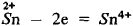and complex molecules and ions that contain atoms with intermediate oxidation numbers, for example,Carbon and carbon monoxide are widely used in industry and engineering to reduce metals from oxides:

ZnO + C = Zn + CO
FeO + CO = Fe + CO2

Sodium sulfite (Na2SO3) and sodium hydrosulfite (NaHSO3) are commonly used in photographic and dyeing processes, while metallic sodium and free hydrogen serve as reducing agents in the purification of metals:

TiCL4 + 4Na = Ti + 4NaCI
GeO2 + 2H2 = Ge + 2H2O

Oxidizing agents can be neutral nonmetal atoms, in particular, halogens and oxygen, and positively charged metal ions with higher oxidation numbers, for example, Sn4+ + 2ē = Sn2+. They can also be complex ions and molecules that contain atoms with higher oxidation numbers, for example,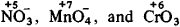The important commercial oxidizing agents include oxygen, which is particularly useful in metallurgy. They also include ozone, nitric acid, hydrogen peroxide, potassium permanganate, calcium hypochlorite, and chromic and dichromic acids with their corresponding salts. The strongest oxidizing agent is an electric current, in which case oxidation takes place at the anode.

As a general rule for selecting coefficients in oxidation-reduction equations, the number of electrons given up by the reducing agent should equal the number of electrons accepted by the oxidizing agent. Coefficients can be selected by the electron-transfer method or by the electron-ion method. In the electron-transfer method, the number of electrons donated and accepted is calculated according to the oxidation numbers of the elements before and after the reaction has taken place. For example,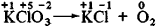Here,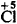is the oxidizing agent and O is the reducing agent, as shown in the following half-reactions: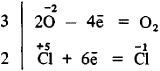In accordance with the general rule, the number of electrons given up is set equal to the number of electrons gained, and the derived values are substituted in the original equation:

2KClO3 = 2KC1 + 3O2

Using the ion-electron method, the reaction is expressed in accordance with the general rules that govern the formulation of ionic reactions; that is, strong electrolytes are represented as ions, while nonelectrolytes, weak electrolytes, gases, and precipitates are designated as molecules. Ions that remain unchanged during the reaction process are not included. For example,

KMnO4 + KI + H2SO4
K2SO4 + I2 + MnSO4 + H2O

In ionic form, this equation would be written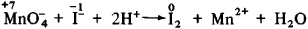Once the oxidation numbers have been computed, the oxidizing and reducing agents are determined and the half-reactions for the oxidation-reduction reaction are formulated:Since O atoms are present on the left side of the second equation and not on the right, the equation must be balanced. The excess O atoms are bonded to H2O molecules by the H+ ions in the acidic reaction medium: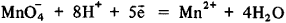As in the electron-transfer method, the next step is to determine coefficients for the half-reactions in order to balance the electrons; these coefficients would be 5 and 2 for the two half-reactions 2I- — 2ē = I2 and MnO4- → Mn2+, respectively. The final equation then becomesThe derived coefficients are substituted in the original equation:

2KMnO4 + 10KI + 8H2SO4 = 6K2SO4 + 5I2 + 2MnSO4 + 8H2O

A similar procedure is used to formulate oxidation-reduction equations in alkaline medium, the difference being that OH-ions are substituted for H+ ions in the half-reactions. Thus, the pH nature of the reaction medium—acid, alkaline, or neutral— is taken into account when using the ion-electron balancing method, since pH has a substantial effect on both the course of the oxidation-reduction reaction and the resultant products. For example, the equilibrium of the reaction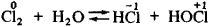shifts to the left in acid medium and to the right in alkaline medium. The strong oxidizing agent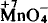ion reduces to Mn2+ in acid medium, to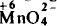in alkaline medium, and to the molecule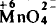in neutral medium.

### REFERENCES

Kudriavtsev, A. A. Sostavlenie khimicheskikh uravnenii. Moscow, 1968. Khimiia: Kurs dlia srednei shkoly, 2nd ed. Moscow, 1972. Chapter 12. (Translated from English.)
Khimiia: Posobie dlia prepodavatelei srednei shkoly, part 1. Moscow, 1973. Chapter 12. (Translated from English.)

V. K. BEL’SKII

References in periodicals archive ?
Potassium hydroxide (KOH), which has a current usage of approximately 580,000 tons/year in the United States, is typically produced using chlor-alkali oxidation-reduction reactions that involve hazardous co-products and a byproduct of chloride gas.
Swimming Pools: a matter of balance, introduces pH, buffers and oxidation-reduction reactions within the context of managing bacterial levels in swimming pools.
Relate the oxidation-reduction reactions in plants to that in animals

Site: Follow: Share:
Open / Close